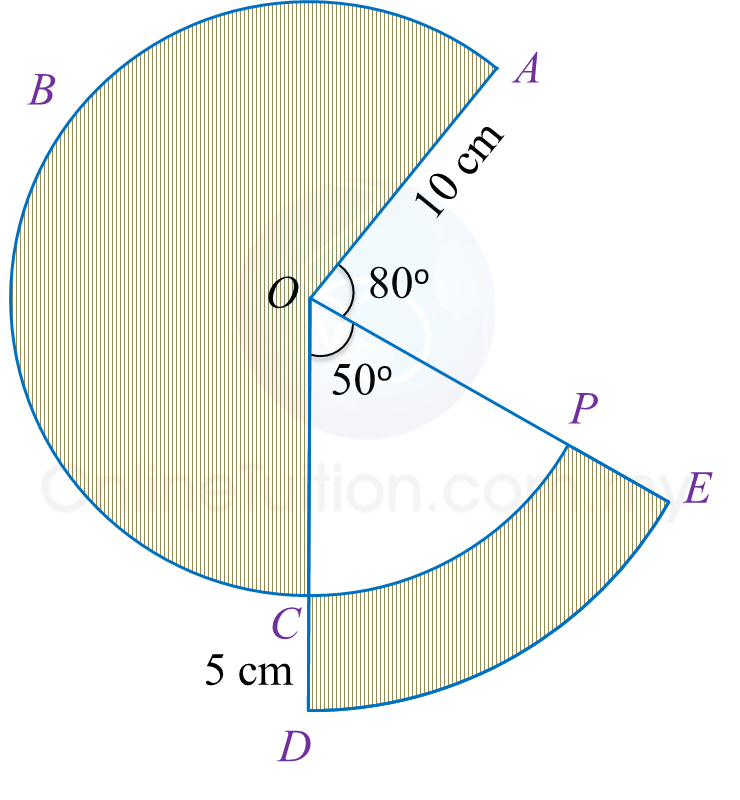# 5.2.6 Circles, PT3 Focus Practice

 
Question 14:
In the Diagram, OKLM is a sector of a circle and OAB is a quadrant of a circle with common centre O.It is given that OA = 8 cm, ∠KOM = 90o and OK : OB = 3 : 2.
Using      π=     22   7        , calculate
(a) the area, in cm2, of the shaded region,
(b) the perimeter, in cm, of the shaded region.

Solution:
 (a)
           OB=OA=8 cm and           OK:OB=3:2                  OK       OB      =    3    2                       OK    8      =    3    2                OK=    3    2      ×8           OK=12                      Area of the shaded region           =(                      270     o                        360     o               ×       22    7       ×       12    2         )(                      90     o                        360     o               ×       22    7       ×    8    2         )           =339    3    7      50    2    7                =289    1    7           cm    2                 

(b)
         Length of arc KLM           =             270    o                   360    o            ×2×       22    7      ×12           =56    4    7       cm                      Length of arc AB           =             90    o                   360    o            ×2×       22    7      ×8           =12    4    7       cm                      Perimeter of shaded region           =Length of arc KLM+Length of            arc AB+MO+OK+OA+OB           =56    4    7      +12    4    7      +12+12+8+8           =109    1    7       cm            

 

Question 15:
Diagram shows sectors OABC and ODE with the common centre respectively.Calculate
(a) the perimeter, in cm, of the whole diagram,
(b) the area, in cm2, of the shaded region.

Solution:
(a)

(b)